Higher courses, Math.Stat.Higher courses, Opt&Syst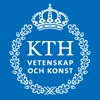Avdelning Matematik### Fall 2008

#### Integration theory

##### KTH, SF 2709, Serguei Shimorin
Course page (in Swedish)For all studies of analysis in higher mathematics it is fundamental to understand the concept of integration. Our intuitive idea of the integral of a function is as the area under its graph, and this can be turned into a formal definition through approximating Riemann sums. This leads to the Riemann integral which works fine in many circumstances, but has its limitations.

One problem with the Riemann integral is that it does not manage functions with too many discontinuities, for example the function f(x) which is 1 if x is rational and 0 if x is irrational cannot be integrated. Morally the integral should have the value zero since the rationals form a countable set which should not contribute to the integral. A more serious problem is that the Riemann integral does not behave nicely when one studies sequences of functions, such as the partial sums of a Fourier series approximating a periodic function. When can one move the limit inside the integral?

In this course we will study the Lebesgue integral, and more general concepts of integrals and measure. Among other things we will see how the above problems are resolved and we will study the important Lp spaces of functions.

The material in this course is fundamental also for the study of probability theory.

#### Topics in mathematics III: Matrix groups

##### KTH, SF 2723, Mats Boij
Course page (in Swedish)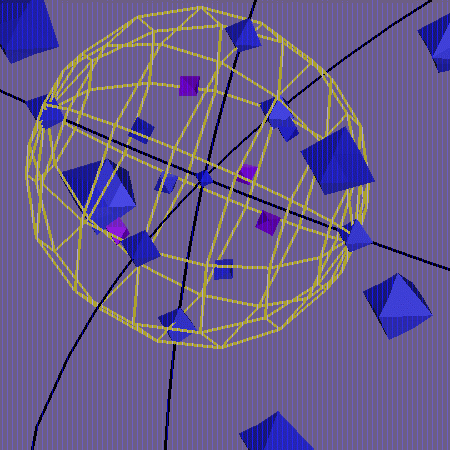There exist many different natural sets of matrices that all have the property that they are closed under multiplication and taking inverses. Among them we find in particular the so-called classical groups and you have certainly come across those in one form or another, whether you realized it or not. They are the general linear group Gln, the special linear group Sln, the ortogonal group On, the special orthogonal group SOn, and the symplectic group Spn.

During the course we will discuss a number of important concepts from 19th and 20th century mathematics that will help us to understand how the classical groups look like. We will use methods from algebra, topology, differential geometry and algebraic geometry. The idea is that matrix groups, which themselves are of great interest in mathematics and physics, will serve as a tool to understand the abstract concepts that are needed to study them.

#### Chaotic dynamical systems

##### KTH, SF 2720, Michael Benedicks
Course page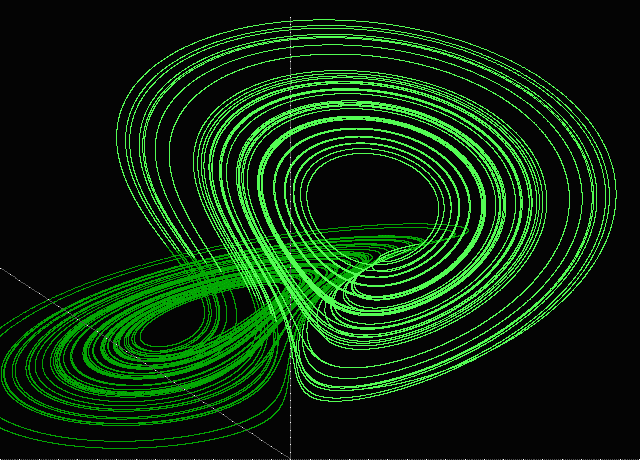For a number of years now, chaotic dynamical systems have received a lot of scientific attention. One aspect is chaos, fractals, etc., often illustrated with the fantastic pictures -- the Mandelbrot set, Julia sets, etc. -- that computer simulations of iterations of complex polynomials give rise to.

Another aspect is formed by the so-called "strange attractors", that occur in conjunction with computer simulations of ordinary differential and difference equations. Some of the best known mathematical experiments were carried out by the meteorologist E. Lorenz and the astronomer M. Hénon, and here at the department precisely these models have been studied rigorously and chaotic behaviour was proved for them. D. Ruelle and F. Takens have proposed that turbulent phenomena might at least partially be explained via strange attractors.

The physicist M. Feigenbaum made the fundamental discovery that many systems first go through a characteristic period doubling and then behave in a random (chaotic) way, even though they are deterministic. Later, one has shown that such period doublings occur in liquid helium flow.

From a mathematical viewpoint, the course is quite special. On a relatively elementary level, one obtains insight in phenomena that lie quite close to current research. One or two computer experiments will probably be part of the course. However, the course's main emphasis will be on the mathematical theory, which in itself has a long history with names such as Poincaré, Fatou, Birkhoff, and Smale, and which lately has developed quickly, partly in symbiosis with computer experiments.

#### Elementary differential geometry

##### SU, MM8010, Andrzej Szulkin
Course page (in Swedish)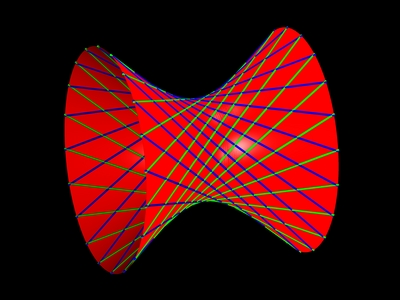In this course we study curves and surfaces in three-dimensional space. This subject has the beauty that one can start from knowing only calculus of several variables, and reach many deep and interesting facts.

The main new concept is that of curvature. In mathematics curvature appears in many different forms, with the common property that it measures how much an object differs from being flat. For example an ordinary sphere has constant positive curvature, and the curvature becomes larger as the radius is decreased. A linear plane has zero curvature, but what would a surface of negative curvature be? Are there closed surfaces of zero or negative curvature?

The most important result covered in the course is the Gauss-Bonnet theorem. This relates the curvature of a surface to a topological quantity (the Euler characteristic). This is a prototype of many results in mathematics where geometry (which is computed locally, at a microscopic level) is related to global topology (which can only be seen by viewing the entire space).

(This course is followed by SF2722 Differential geometry at KTH.)

#### Algebra IV

##### SU, MM8004, Ralf Fröberg
Course page (in Swedish)

The course is a natural continuation of Algebra III at SU, where groups, rings and fields are introduced. These notions are developed further and new concepts are introduced as well. The material, which in itself is abstract in nature, finds applications in several areas of mathematics and is of fundamental importance in algebraic geometry and algebraic topology. Topics include the tensor product, symmetric and exterior algebras, chain conditions, modules, Jordan's normal form, simple and semisimple algebras. (The course is quite similar, but not identical, to SF2706 at KTH, which will not be given 2008/09.)

#### Analytic functions 2

##### SU, MM8007
Course page (in Swedish)

The course is a natural continuation of Analytic functions I at SU. Some of the topics treated are: power series, analytic functions as conformal maps, Möbius transformations with applications, elliptic functions, modular forms, Cauchy's integral formula and the Cauchy integral theorem with applications, the argument principle, and the Riemann mapping theorem or the Dirichlet problem.

### Spring 2009

#### Topology

##### KTH, SF 2721, Björn Gustafsson

Room 3733 (KTH), Tuesdays at 13-15. Course start: January 27

Course page (in Swedish)Topology is the study of spaces from an abstract viewpoint. One is interested both in the fine structure of a space and in global features such as the number of holes. A fundamental concept is that of a continuous function, or continuous map, and the goal is to understand what properties such a map can have without using ideas like distance or derivative.

For instance, it might seem obvious that a simple closed curve in the plane divides the plane into an "inside" and an "outside" region. This observation is correct, but to really prove it assuming only that the curve is continuous is not an easy task. In fact, this was a hard problem for a long time, studied by many mathematicians in the 19th century. In the course we will see a proof of this "Jordan curve theorem", and other results such as the "Ham sandwich theorem" (one can always divide a three layer sandwich into two equal pieces with just one cut) and the "Hairy ball theorem" (one cannot comb a hedgehog).

A classical example in topology is that, in a world of perfect rubber, a coffee cup cannot be distinguished from a doughnut, but is fundamentally different from a ball. What does this observation mean? And how can one turn it into computable mathematics? The answer, perhaps surprisingly, involves group theory and abstract algebra. In the course we will see how to find and classify all two-dimensional surfaces. The doughnut and the ball are two of them.

#### Topics in mathematics IV: Prime numbers

##### KTH, SF 2724, Anders Karlsson

Room 3733 (KTH), Thurdays at 10-12. Course start: January 22

Course page (in Swedish)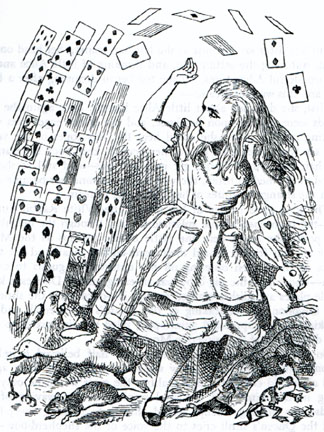Everybody knows what a prime number is and that there are infinitely many of them; this course will go further. Some of the topics that will be discussed: the prime number theorem (how the number of prime numbers less than x grows with x); famous unsolved problems (such as the Riemann hypothesis, the Goldbach conjecture, and whether there are infinitely many prime twins) and results related to these; a discussion of the abc conjecture, how it implies Fermat's last theorem (proved by Andrew Wiles in 1994), and a quick proof of the polynomial version of the conjecture; famous solved problems, such as Dirichlet's theorem and a discussion of the recent theorem of Green and Tao (both results deal with prime numbers in arithmetic progressions). The proof of the prime number theorem requires some complex analysis, but the rest of the material is largely elementary. The course will provide an opportunity to learn about fundamental results and open questions regarding prime numbers and to appreciate the beauty and unity of mathematics.

#### Differential geometry

##### KTH, SF 2722, Mattias Dahl

Room 3733 (KTH), Tuesdays at 10-12. Course start: January 27

Course page (in Swedish)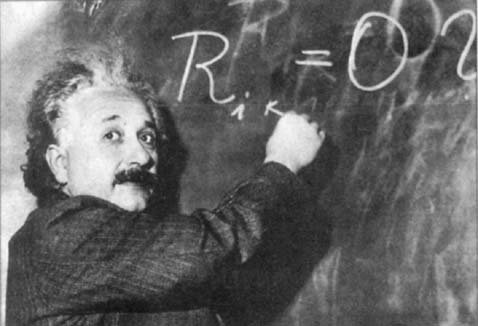In this course, differential geometry is developed from an abstract viewpoint. The central objects of study are differentiable manifolds, which are higher-dimensional generalizations of curves and surfaces, without the restriction of being embedded in some Rn. The essential idea is to study analysis in a coordinate-free fashion. The derivative of a smooth map is "the best linear approximation" at a point, and in calculus one has seen how to compute it as a matrix of partial derivatives. But what does this mean if we have no particular coordinates in which to compute partial derivatives?

Along the way we will see proper definitions of things like vector and tensor fields, and we will see how to formulate and prove results from vector analysis in a general setting. This coordinate-free machinery is fundamental for many physical theories such as classical mechanics and general relativity.

A manifold has a local structure which allows us to do analysis, but on a global scale it can have interesting topological features. We will see how analysis and topology come together in the notion of the degree of a smooth map and also in Morse theory.

The concept of curvature appears when one introduces a Riemannian metric on a manifold. In the course we will see how to define distances, covariant derivatives, geodesics, and the curvature tensor, and what they mean for the local geometry of a manifold.

(This course follows naturally "Elementary differential geometry" at Stockholm University, and it is recommended to have taken this course. A good knowledge of calculus of several variables and the inverse and implicit function theorems is an important prerequisite.)

#### Topics in mathematics V: Clifford algebras, geometric algebra, and applications

##### KTH, SF 2725, Lars Svensson and Douglas Lundholm

Room 3721 (KTH), Thurdays at 15-17. Course start: January 29

Course page (in Swedish)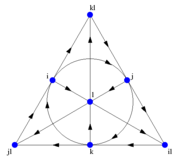It is well known that the complex numbers form a powerful tool in the description of plane geometry. The geometry of 3-dimensional space is traditionally described with the help of the scalar product and the cross product. However, already before these concepts were established, Hamilton had discovered the quaternions, an algebraic system with three imaginary units which makes it possible to deal effectively with geometric transformations in three dimensions.

Clifford originally introduced the notion nowadays known as Clifford algebra (but which he himself called geometric algebra) as a generalization of the complex numbers to arbitrarily many imaginary units. The conceptual framework for this was laid by Grassmann already in 1844, but it is only now that one has fully begun to appreciate the algebraisation of geometry in general that the constructions of Clifford and Grassmann result in. Among other things, one obtains an algebraic description of geometric operations in vector spaces such as orthogonal complements, intersections, and sums of subspaces, which gives a way of proving geometric theorems that lies closer to the classical synthetic method of proof than for example Descartes's coordinate geometry. This formalism gives in addition a natural language for the formulation of classical physics and mechanics.

The best known application of Clifford algebras is probably the "classical" theory of orthogonal maps and spinors which is used intensively in modern theoretical physics and differential geometry.

This course will run as a graduate course, but it will also be possible to take it as an advanced level course.

#### Elementary algebraic geometry

##### SU, MM8013

Room 306 (SU), Mondays at 13-15. Course start: January 19

Course page (in Swedish)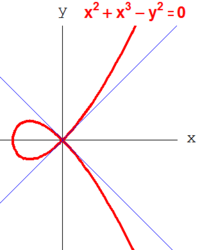Algebraic geometry is the study of the solutions of systems of polynomial equations. Due to the special form of the equations, the solution spaces, called algebraic varieties, are not as 'flexible' as for example differentiable manifolds, but they carry a lot of structure. For example, deep results have been obtained that relate the solution spaces of the `same' system of equations over different ground fields to each other.

This course introduces basic algebro-geometric notions such as affine and projective algebraic varieties, dimension, nonsingularity, and maps between algebraic varieties. Several concrete examples, such as algebraic curves and surfaces, will be discussed as well.

#### Galois theory

##### SU, MM8005

Room 306 (SU), Thurdays at 13-15. Course start: January 22

Course page (in Swedish)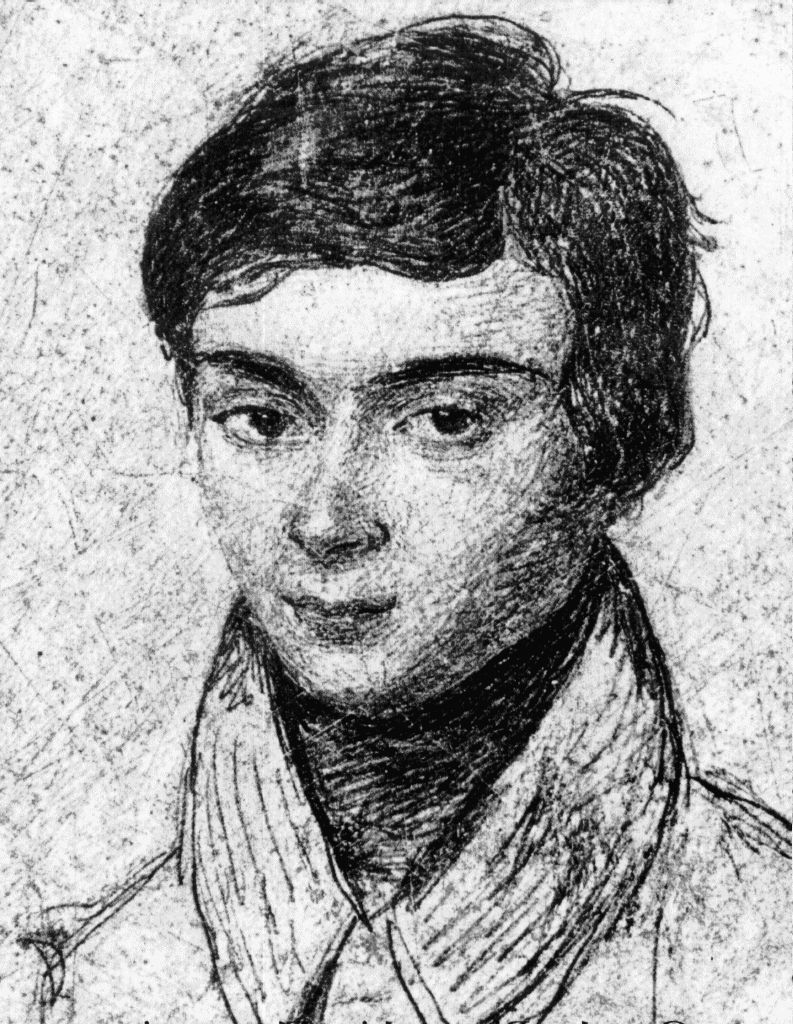Galois theory is a beautiful and fundamental part of algebra dealing with field extensions and field automorphisms. The main theorem gives a 1-1 correspondence between the subextensions of a given field extension satisfying certain properties and the subgroups of a group of automorphisms associated to the extension.

Galois theory has many applications. Some of the best known applications are the proof of the impossibility of the trisection of a general angle with ruler and compass only and the proof that the solutions of a general algebraic equation of degree five or higher cannot be given only in terms of n-th roots and the basic algebraic operations.

#### Mathematical and computational methods from micro to macro scales

##### KTH, Anders Szepessy

Room 4523 (KTH), Tuesdays at 10-12. Course start: January 27

Course page

#### Optimal control

##### KTH, SF2852, Ulf Jönsson

Room D34 (KTH), Wednesdays at 10-12. Course start: January 14

Course page (in Swedish)

#### Fördjupning i finansmatematik, fdk

##### SU, Thomas Höglund, Joanna Tyrcha

Room 14 (SU), Tuesdays and Fridays at 9-12. Course start: January 20

Course page

#### Analys av kategoridata, pk

##### SU, Juni Palmgren, Jan-Olov Persson

Room 32 (SU), Misc. days. Course start: March 23

Course page

#### Statistiska modeller, fdk

##### SU, Rolf Sundberg

Room 32 (SU), Tuesdays and Fridays at 9-12. Course start: March 24

Course pageSidansvarig: Webmaster